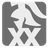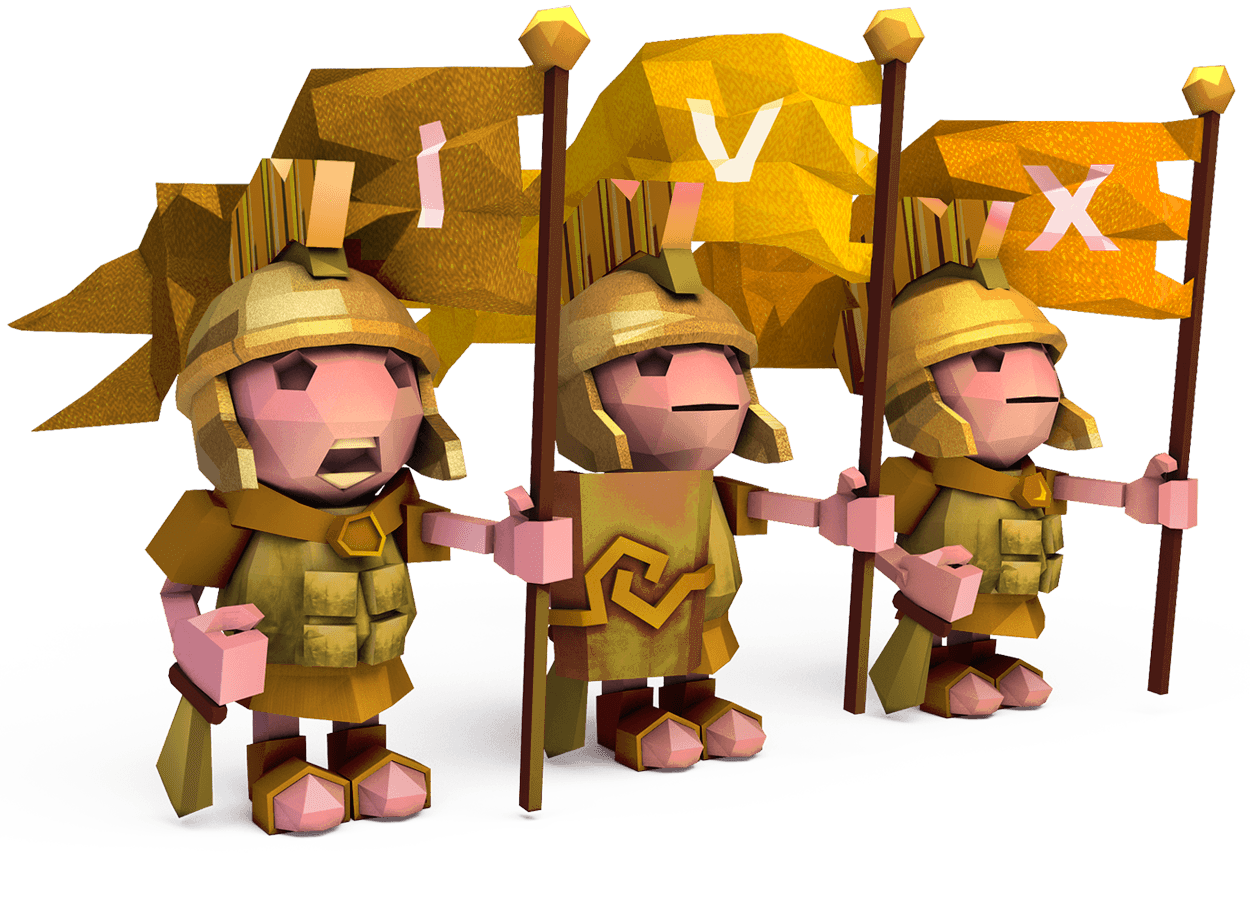Reverse Roman Numerals
Simple
English RUIn the CheckiO mission Roman Numerals you have to convert a decimal number into its representation as a roman number.
Here you have to do the same but the other way around.

You are given a Roman number as a string and your job is to convert this number into its decimal representation.

A valid Roman number, in the context of this mission, will only contain Roman numerals as per the below table and follow the rules of the subtractive notation.
Check this Wikipedia article out for more details on how to form Roman numerals.

NumeralValue
I1 (unus)
V5 (quinque)
X10 (decem)
L50 (quinquaginta)
C100 (centum)
D500 (quingenti)
M1,000 (mille)

Input: A roman number as a string.

Output: The decimal representation of the roman number as an int.

Example:

```reverse_roman('VI') == 6
reverse_roman('LXXVI') == 76
reverse_roman('CDXCIX') == 499
reverse_roman('MMMDCCCLXXXVIII') == 3888```

Precondition:
len(roman_string) > 0
all(char in "MDCLXVI" for char in roman_string) == True
0 < reverse_roman(roman_string) < 4000